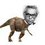# Lets do something meaningless... :P

I was tired solving maths problems , then I stopped for a minute and I thought of doing something meaningless which includes my friend Trevor A (prototype) and the brilliant avatar Trevor B.So what I am going to prove where $TREVOR$ is an integer is that :

$\Large min(TREVOR) = \pm 1$

Proof:

Lets assume that $TREVOR \ A > TREVOR \ B$.

But $1<2 \Rightarrow A

By multiplying both the sides by $TREVOR$ , the inequality sign flips which tells us that:

$\Large min(TREVOR) = -1$

Lets consider another case $TREVOR \ A < TREVOR \ B \\ A < B$

By multiplying both the sides by $TREVOR$ , the inequality sign remains the same which tells us that :

$\Large min(TREVOR) = 1$

Let us consider one more case $TREVOR \ A = TREVOR \ B$

But $A makes it impossible hence , $TREVOR \ A \neq TREVOR \ B$. Hence this case does not hold true.

At last we conclude that $min(TREVOR) = \pm 1$

I hope this made you feel nice in your busy study schedule.Cheers!Note by Nihar Mahajan
5 years ago

This discussion board is a place to discuss our Daily Challenges and the math and science related to those challenges. Explanations are more than just a solution — they should explain the steps and thinking strategies that you used to obtain the solution. Comments should further the discussion of math and science.

When posting on Brilliant:

• Use the emojis to react to an explanation, whether you're congratulating a job well done , or just really confused .
• Ask specific questions about the challenge or the steps in somebody's explanation. Well-posed questions can add a lot to the discussion, but posting "I don't understand!" doesn't help anyone.
• Try to contribute something new to the discussion, whether it is an extension, generalization or other idea related to the challenge.

MarkdownAppears as
*italics* or _italics_ italics
**bold** or __bold__ bold
- bulleted- list
• bulleted
• list
1. numbered2. list
1. numbered
2. list
Note: you must add a full line of space before and after lists for them to show up correctly
paragraph 1paragraph 2

paragraph 1

paragraph 2

[example link](https://brilliant.org)example link
> This is a quote
This is a quote
    # I indented these lines
# 4 spaces, and now they show
# up as a code block.

print "hello world"
# I indented these lines
# 4 spaces, and now they show
# up as a code block.

print "hello world"
MathAppears as
Remember to wrap math in $$ ... $$ or $ ... $ to ensure proper formatting.
2 \times 3 $2 \times 3$
2^{34} $2^{34}$
a_{i-1} $a_{i-1}$
\frac{2}{3} $\frac{2}{3}$
\sqrt{2} $\sqrt{2}$
\sum_{i=1}^3 $\sum_{i=1}^3$
\sin \theta $\sin \theta$
\boxed{123} $\boxed{123}$

Sort by:

LOL , What is this? Are you okay?

- 5 years ago

Yes , I am okay. If you don't understand its ok.Its just a time pass stuff.

- 5 years ago

Kaboobly Doo!

Yeah, Kaboobly Doo Stuff :P XD

- 5 years ago

@Nihar Mahajan, as a continued exercise, prove the following:

$\zeta(\textrm{TREVOR A})=69$

- 5 years ago

Lol. But i don't know zeta function yet. :(

- 5 years ago

Let me enlighten you. The value $\zeta(\textrm{TREVOR A})$ is a special value, unlike the rest of the zeta function values. I suspect that it might be the key to proving the Trevormann hypothesis since it has a real part of $69$ and is still a zero of the Trevormann zeta function.

@Pi Han Goh, I think you should look into this. We have stumbled upon yet another discovery. Shall we uncover it together?

- 5 years ago

LOL...Omg ... if its established , it would be really amazing :P

- 5 years ago

So you're telling me that $\text{TREVOR A}=1.014615241815$?

This might be a new discovery you've had... As it follows the constraint that $min(TREVOR)=\pm1$

- 5 years ago

Should I publish the proof myself in Arxiv?

I also have a maximum upper bound for the A version but I'm lacking a complete proof since I haven't researched on the B version.

- 5 years ago

:3 this actually made me laugh pretty hard. Haha.

But you forgot to consider the case of $TREVOR=0$. In which case the world implodes.

- 5 years ago

Well the proof is not complete, you need to prove that A <B ...... 😛😛

- 5 years ago

I have proved it. A=1 , B=2 so , A < B . :P

- 5 years ago

How can u assume that A = 1 & B= 1?? :P

- 5 years ago

@Harsh Shrivastava , He assumed the values of A and B to Be their "Place" values in the alphabet :P. Also, Nice Proof, @Nihar Mahajan XD

- 5 years ago

ikr , XD :P

- 5 years ago

@Trevor Arashiro @Trevor B. Do read this note. :P

- 5 years ago